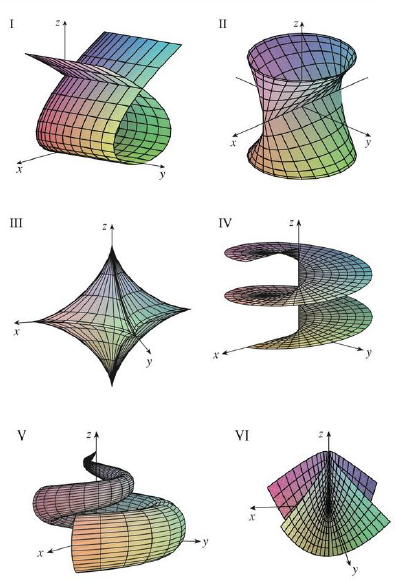Chapter 16.6, Problem 16E

Chapter
Section
Textbook Problem

Match the equations with the graphs labeled I–VI and give reasons for your answers. Determine which families of grid curves have u constant and which have v constant.16. x = (1 − u)(3 + cos v) cos 4πu, y = (1 − u)(3 + cos v) sin 4πu, z = 3u + (1 − u) sin vTo determine

To match: The parametric equations x=(1u)(3+cosv)cos4πu,y=(1u)(3+cosv)sin4πu,z=3u+(1u)sinv with the graphs labeled I-VI in the textbook and to explain the reason for the combination.

Explanation

Given data:

Write the given parametric equations as follows.

x=(1u)(3+cosv)cos4πu,y=(1u)(3+cosv)sin4πu,z=3u+(1u)sinv

The graphs are labeled in the textbook from I to VI.

Determination of grid curves when u is constant:

Consider the parameter u=0 .

Substitute 0 for u in the parametric equations as follows.

x=(10)(3+cosv)cos4π(0),y=(10)(3+cosv)sin4π(0),z=3(0)+(10)sinvx=(3+cosv)(1),y=(3+cosv)(0),z=0+sinvx=3+cosv,y=0,z=sinv

From the expression x=3+cosv,y=0,z=sinv , it is clear that the equation represents the circle with radius 1 in the xz-plane centered at the point (3,0,0) .

Consider the parameter u=12 .

Substitute 12 for u in the parametric equations,

x=(112)(3+cosv)cos4π(12),y=(112)(3+cosv)sin4π(12),z=3(12)+(112)sinvx=(12)(3+cosv)(1),

Still sussing out bartleby?

Check out a sample textbook solution.

See a sample solution

The Solution to Your Study Problems

Bartleby provides explanations to thousands of textbook problems written by our experts, many with advanced degrees!

Get Started

Find the median for the following set of scores: 1, 9, 3, 6, 4, 3, 11, 10

Essentials of Statistics for The Behavioral Sciences (MindTap Course List)

In Exercises 58, evaluate the expression. 6. |51243|

Applied Calculus for the Managerial, Life, and Social Sciences: A Brief Approach

ln |2x + 1| + C 2 ln |2x + 1| + C

Study Guide for Stewart's Single Variable Calculus: Early Transcendentals, 8th

The polar form for the graph at the right is:

Study Guide for Stewart's Multivariable Calculus, 8th